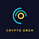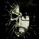1732 visualizzazioni
1732
Wavy Tunnel Trading Method
study(title="Bungee", shorttitle="Bungee")

OverBought = hline(80)
OverSold = hline(20)

length1 = input(76, minval=1), smoothK1 = input(4, minval=1), smoothD1 = input(4, minval=1)
k1 = sma(stoch(close, high, low, length1), smoothK1)
plot(k1, color=orange)

length2 = input(3, minval=1), smoothK2 = input(3, minval=1), smoothD2 = input(3, minval=1)
k2 = sma(stoch(close, high, low, length2), smoothK2)
plot(k2, color=green)

length3 = input(16, minval=1), smoothK3 = input(3, minval=1), smoothD3 = input(3, minval=1)
k3 = sma(stoch(close, high, low, length3), smoothK3)
plot(k3, color=blue)

length4 = input(8, minval=1), smoothK4 = input(3, minval=1), smoothD4 = input(3, minval=1)
k4 = sma(stoch(close, high, low, length2), smoothK4)
plot(k4, color=red)

length5 = input(14, minval=1)
upper = highest(length5)
lower = lowest(length5)
WP = 100 * (upper - close) / (upper - lower)
plot(WP, color=purple)

length6 = input(2, minval=1)
up = rma(max(change(close), 0), length6)
down = rma(-min(change(close), 0), length6)
RSI = down == 0 ? 100 : up == 0 ? 0 : 100 - (100 / (1 + up / down))
plot(RSI, color=black)Here you make mistake.

length5 = input(6, minval=2)
upper = highest(length5)
lower = lowest(length5)
WP = 100 + (-100 * (upper - close) / (upper - lower))
plot(WP, color=purple)
RispondiHI,Friend
thank you for your sharing "bungee",i like it very much,But your indicators have a small error(length5 is error),can you help me to fix it? thank you very much.

Best regards
Rispondijackvmk
It's really not possible to explain how this works with the Elliot Wave in a forum, because it's extremely complicated. This type of training has been done online classes. It's called the "Wavy Tunnel Trading System". I'm not trying to avoid your question, but the class is several months of online webinars that can't be explained in a few messages. Your welcome to use my indicators though.
Rispondi